## Definition Of Median Of A Triangle

Median of a Triangle is a line segment joining a vertex of the triangle to the midpoint of the opposite side of the triangle.

### More About Median of a Triangle

The three medians of a triangle are concurrent and the concurrent point is called the centroid of the triangle.
The centroid of a triangle divides the medians in the ratio 2:1, i.e. the length of the median from the vertex to the centroid is twice the length of the median from the centroid to the opposite side of the vertex.

### Examples of Median of a Triangle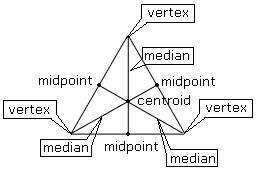### Solved Example on Median of a Triangle

#### Ques: Identify the median of a triangle.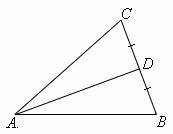##### Choices:

A.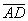B.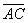C.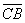D.
Step 2:is the only median drawn from the vertex A to the midpoint of the opposite side of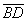.
Step 3: So,is the median of a triangle.